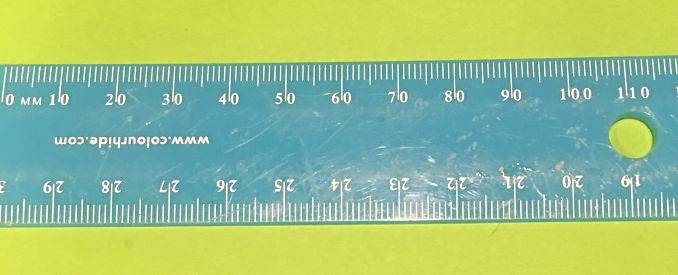# Definition of Milli-Milli- is a prefix that means $\dfrac{1}{1000}$ (one thousandth).

For example,

• A millilitre is $\dfrac{1}{1000}$th of a litre.
• A milligram is $\dfrac{1}{1000}$th of a gram.
• A millimetre is $\dfrac{1}{1000}$the of a metre.
• A millipede doesn't really have $1000$ legs, although it does have a lot! It can have up to 200 pairs of legs.

### Description

The aim of this dictionary is to provide definitions to common mathematical terms. Students learn a new math skill every week at school, sometimes just before they start a new skill, if they want to look at what a specific term means, this is where this dictionary will become handy and a go-to guide for a student.

### Audience

Year 1 to Year 12 students

### Learning Objectives

Learn common math terms starting with letter M

Author: Subject Coach
You must be logged in as Student to ask a Question.